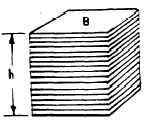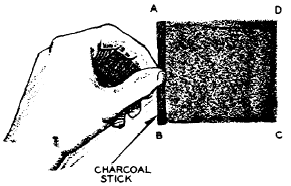Custom SearchPRISMS The solid shown in figure 18-10 is a PRISM. A prism is a solid with three or more lateral faces which intersect in parallel lines. Types of Prisms The name of a prism depends upon its base polygons. If the bases are triangles, as in figure 18-10, the figure is a TRIANGULAR prism. A RECTANGULAR prism has bases which are rectangles. If the bases of a prism are perpendicular to the planes forming its lateral faces, the prism is a RIGHT prism. A PARALLELEPIPED is a prism with parallelograms for bases. Since the bases are parallel to each other, this means that they cut the lateral faces to form parallelograms. There parallelograms. If a parallelepiped is a right prism, and if its bases are rectangles, it is a rectangular solid. A CUBE is a rectangular solid in which all of the six rectangular faces are squares. Parts of a Prism The parts of a prism are shown in figure 18-10. The line formed by the joining of two faces of a prism is an EDGE. If the two faces forming an edge are lateral faces, the edge thus formed is a LATERAL EDGE. Surface Area and Volume The SURFACE AREA of a prism is the sum of the areas of all of its faces, including the bases. The VOLUME of a prism may be considered as the sum of the volumes of many thin wafers, each having a thickness of one unit and a shape that duplicates the shape of  the base.Figure 18-11. -Volume of a prism. The wafers which comprise the prism in figure 18-11 all have the same area, which is the area of the base. Therefore, the volume of the prism is found by multiplying the area of the base times the number of wafers. Since each wafer is 1 inch thick, the number of wafers is the same as the height of the prism in inches. The resulting formula for the volume of a prism, using B to represent the area of the base and h to represent the height, is as follows: V = Bh When a prism has lateral edges which are not perpendicular to the bases, the height of the prism is the perpendicular distance between the bases. (See fig. 18-12.) The formula for the volume remains the same, even though’ the prism is no longer a right prism.Figure 18-12.-Height of a prism which is not a right prism. CIRCULAR CYLINDERS Any surface may be considered as the result of moving a straight line in a direction at right angles to its length. For example, suppose that the stick of charcoal in figure 18-13 is moved from position CD to position AB by dragging it across the paper. The broad mark made by the charcoal represents a plane surface. The surface is said to be "generated" by moving line AB.Figure 18- 13 .-Surface generated by a moving line. The movement of the line in figure 18-13 may be controlled by requiring that its lower end trace a particular path. For example, if line AB moves so as to trace an ellipse as in figure 18-14 (A), a cylindrical surface is genure 18-14 (B), is an elliptical cylinder. Any line in the surface, parallel to the generating line, such as CD or EF in figure 18-14 (B), is an ELEMENT of the cylinder. If the elements are perpendicular to the bases, the cylinder is a RIGHT CYLINDER. If theFigure 18-14.-(A) Line generating a cylinder; (B) elliptical cylinder; (C) circular cylinder. b CYLINDER. Figure 18-14 (C) illustrates a right circular cylinder. Line O-O’, joining the centers of the bases of a right circular cylinder, is the AXIS of the cylinder.